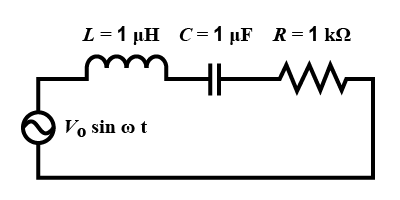QuestionAnswers

# In the circuit shown, $L\quad =\quad 1\mu H$ , $C\quad =\quad 1\mu F$ and $R\quad =\quad 1k\Omega$. They are connected in series with an a.c. source $V\quad ={ V }_{ 0 }\sin { \omega t }$ as shown. Which of the following options is/are correct?A.)At $\omega \quad >>{ 10 }^{ 6 }\quad rad.{ r }^{ -1 }$, the circuit behaves like a capacitorB.)At $\omega \sim 0$ the current flowing through the circuit becomes nearly zero.C.)The current will be in phase with the voltage if $\omega ={ 10 }^{ 4 }rad.{ s }^{ -1 }$D.)The frequency at which the current will be in phase with the voltage is independent of R.Hint: Use formula for impedance in series LCR circuit. Calculate resonance frequency using impedance formula. This frequency is independent of resistance. Then, use the formula for current in LCR series and let frequency be equal to zero. Thus, current becomes zero.

Formula used:
$Z\quad =\quad R\quad +\quad j\omega L\quad -\dfrac { j }{ \omega C }$
${ \omega }_{ 0 }=\dfrac { 1 }{ \sqrt { LC } }$
$I\quad =\quad \dfrac { V }{ \sqrt { { R }^{ 2 }+\quad { \left( \omega L\quad -\dfrac { 1 }{ \omega C } \right) }^{ 2 } } }$

When L, R and C are connected in series, impedance is given by,
$Z\quad =\quad R\quad +\quad j\omega L\quad -\dfrac { j }{ \omega C }$ …(1)

Resonant frequency in series LCR circuit is given by,
${ \omega }_{ 0 }=\dfrac { 1 }{ \sqrt { LC } }$
Substituting values in above equation we get,
${ \omega }_{ 0 }=\dfrac { 1 }{ \sqrt { { 10 }^{ -6 }\times { 10 }^{ -6 } } }$
$\therefore \quad { \omega }_{ 0 }=\sqrt { { 10 }^{ -12 } }$
$\therefore \quad { \omega }_{ 0 }=\quad { 10 }^{ 6 }rad$
This obtained value is independent of R.
Now, current I in series LCR circuit is given by,
$I\quad =\quad \dfrac { V }{ \sqrt { { R }^{ 2 }+\quad { \left( \omega L\quad -\dfrac { 1 }{ \omega C } \right) }^{ 2 } } }$
At $\omega \sim 0$, above equations become,
$I\quad =\quad \cfrac { V }{ \infty }$
$\therefore \quad I\quad =\quad 0$
Thus, the current flowing through the circuit becomes zero.

At $\omega \sim 0$ the current flowing through the circuit becomes nearly zero and the frequency at which the current will be in phase with the voltage is independent of R respectively.

So, the correct answer is “Option B and D”.

Note:
When current is in phase with voltage, Impedance (I) becomes equal to resistance (R).
Therefore, the equation. (1) becomes,
$j\omega L\quad =\quad \dfrac { j }{ \omega C }$
$\therefore \quad { \omega }^{ 2 }=\quad \dfrac { 1 }{ LC }$
$\therefore \quad { \omega }=\quad \dfrac { 1 }{ \sqrt { LC } }$
AC Voltage Applied to Series LCR CircuitIntegrated CircuitCBSE Class 12 Physics Nuclei FormulaResistors in Series and ParallelCBSE Class 12 Physics Atoms FormulaCBSE Class 12 Physics Electromagnetic Waves FormulaCBSE Class 12 Physics Electromagnetic Induction FormulaCBSE Class 12 Physics Wave Optics FormulaCBSE Class 12 Physics Alternating Current FormulaCBSE Class 12 Physics Current Electricity FormulaImportant Questions for CBSE Class 12 PhysicsImportant Questions for CBSE Class 12 Physics Chapter 12 - AtomsImportant Questions for CBSE Class 11 Physics Chapter 12 - ThermodynamicsImportant Questions for CBSE Class 12 Physics Chapter 13 - NucleiImportant Questions for CBSE Class 12 Physics Chapter 15 - Communication SystemsImportant Questions for CBSE Class 12 Physics Chapter 10 - Wave OpticsImportant Questions for CBSE Class 12 Physics Chapter 3 - Current ElectricityImportant Questions for CBSE Class 12 Physics Chapter 8 - Electromagnetic WavesImportant Questions for CBSE Class 12 Physics Chapter 7 - Alternating CurrentImportant Questions for CBSE Class 12 Physics Chapter 6 - Electromagnetic InductionPhysics Question Paper for CBSE Class 12 - 2016 Set 1 CCBSE Class 12 Physics Question Paper 2020Previous Year Question Paper for CBSE Class 12 PhysicsPrevious Year Question Paper for CBSE Class 12 Physics - 2015Previous Year Question Paper for CBSE Class 12 Physics - 2018Previous Year Question Paper for CBSE Class 12 Physics - 2014Previous Year Question Paper for CBSE Class 12 Physics - 2013Previous Year Question Paper for CBSE Class 12 Physics - 2019Previous Year Physics Question Paper for CBSE Class 12 - 2017Previous Year Question Paper for CBSE Class 12 Physics - 2016 Set 1 SNCERT Solutions for Class 12 Physics Chapter 12 Atoms in HindiNCERT Solutions for Class 12 Physics in HindiNCERT Solutions for Class 12 Physics Chapter 15 Communication Systems in HindiNCERT Solutions for Class 12 Physics Chapter 2 Electrostatic Potential and Capacitance in HindiNCERT Solutions for Class 12 Physics Chapter 13 Nuclei in HindiNCERT Solutions for Class 12 Physics Chapter 9 Ray Optics and Optical Instruments in HindiNCERT Solutions for Class 12 Physics Chapter 8 Electromagnetic Waves In HindiNCERT Solutions for Class 12 Physics Chapter 5 Magnetism And Matter In HindiNCERT Solutions for Class 12 Physics Chapter 1 Electric Charges and Fields In HindiNCERT Solutions for Class 12 Physics Chapter 14 Semiconductor Electronic: Material, Devices And Simple Circuits in Hindi